# Birkhoff factorization

(diff) ← Older revision | Latest revision (diff) | Newer revision → (diff)

Birkhoff decomposition

Traditionally, Birkhoff factorization refers to representations of an invertible matrix-functionon the unit circleof the form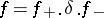, whereare the boundary values of invertible matrix-functions holomorphic inside (respectively, outside)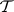, and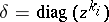is a diagonal matrix-function with some integers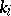as exponents.

More precisely, letbe the standard decomposition of the Riemann sphere, whereis the unit disc and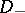is the complementary domain containing the point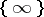. For any domains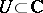,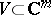, denote by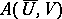the set of all continuous mappings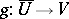which are holomorphic inside. A classical theorem of G. Birkhoff [a1] yields that, for any Hölder-continuous matrix-function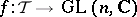, there exist integers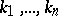and functions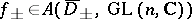, with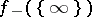equal to the identity matrix, such thaton, where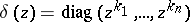. The integers, called the partial indices of, are uniquely determined up to the order and define an interesting decomposition of the space of matrix-functions (cf. Birkhoff stratification). Their sum is an important topological invariant, equal to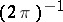times the increment of the argument of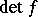along[a2].

Existence and analytic properties of such factorizations have been established for various classes of matrix-functions, [a2]. For a rational, the partial indices and factors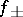are effectively computable [a2]. Similar results are available for factorizations of the form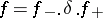.

The Birkhoff theorem is closely related to a number of fundamental topics in algebraic geometry, complex analysis, the theory of differential equations, and operator theory. In particular, it is equivalent to Grothendieck's theorem on decomposition of holomorphic vector bundles over the Riemann sphere [a3]. It is also of fundamental importance for the theory of singular integral equations [a4], Riemann–Hilbert problems and other boundary value problems for holomorphic functions [a5], as well as for the theories of Wiener–Hopf [a6] and Toeplitz operators [a7]; see also [a13].

An extended concept of Birkhoff factorization has recently (1986) emerged in the geometric theory of loop groups [a8]. It turned out that such factorizations are available for various classes of loops on any compact Lie group, with diagonal matricesreplaced by homomorphisms ofinto a maximal torus of. The classical Birkhoff factorization is obtained by taking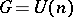, the unitary group. The approach of [a8] has stimulated the geometric study of loop groups [a9] and has permitted one to generalize the classical theory of Riemann–Hilbert problems [a10]. The geometric approach to Birkhoff factorization, developed in [a8], has also interesting applications in the theory of completely integrable models [a11] and-theory [a12].

How to Cite This Entry:
Birkhoff factorization. Encyclopedia of Mathematics. URL: http://encyclopediaofmath.org/index.php?title=Birkhoff_factorization&oldid=16319
This article was adapted from an original article by G. Khimshiashvili (originator), which appeared in Encyclopedia of Mathematics - ISBN 1402006098. See original article